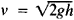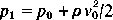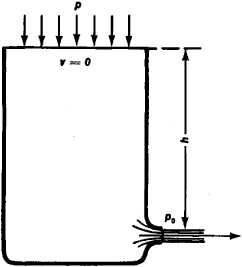# Bernoulli's Equation

Also found in: Dictionary, Medical.

## Bernoulli’s Equation

a basic equation of hydrodynamics that connects (for a steady flow) the velocity of a flowing fluid v, its pressure ρ, and the height h of the location of a small volume of fluid above a reference plane. Bernoulli’s equation was derived by D. Bernoulli in 1738 for a small stream of ideal, incompressible fluid of constant density ρ which is under the influence only of gravitational force. In this case, Bernoulli’s equation takes the form

v2/2 + p/p + gh = constant

where g is the acceleration of gravity. If this equation is multiplied by ρ, then the first term will represent the kinetic energy of a unit volume of fluid, and the other two terms will represent the potential energy, one part of which is determined by the force of gravity (the last term of the equation) and the other part by the pressure ρ. Bernoulli’s equation in such a form expresses the law of conservation of energy. If one form of energy—for example, kinetic—is increasing along the fluid stream, then the potential energy decreases by the same amount. Therefore, for example, upon reduction (narrowing) of the stream flowing in a tube, when the flow velocity increases (since in the same time period the same quantity of fluid passes through the narrowed cross section as through the larger cross section), the pressure in the tube decreases correspondingly. (The principle of operation of the venturi tube is based on this phenomenon.)

A number of important consequences follow from Bernoulli’s equation. For example, in the discharge of a fluid from an open vessel under the influence of gravity (see Figure 1), it follows from Bernoulli’s equation that v 2/2g = h, or—that is, the velocity of the fluid at the outlet aperture is the same as that of fluid particles falling freely from the height h.

If a uniform flow of fluid with velocity v0 and pressure p0 encounters an obstacle along its path (see Figure 2), then a backwater—a retardation of the flow—arises directly in front of the obstacle; in the center of the backwater region, at the critical point, the flow velocity is equal to zero. From Bernoulli’s equation, it follows that the pressure at the critical point is. The pressure increment at this point, equal to, is called the dynamic pressure orFigure 1. Discharge from an open vessel

the velocity head. In a stream of real fluid, its mechanical energy is not conserved along the flow, but is used up in doing frictional work and dissipated in the form of thermal energy; consequently, in the application of Bernoulli’s equation to a real fluid, it is necessary to take into account the losses caused by drag.Figure 2. Flow around an obstacle

Bernoulli’s equation is of great importance in hydraulics and technical hydrodynamics: it is used in pipeline and pump calculations, in the solution of problems involving filtration, and so on. Bernoulli’s equation for a medium with variable density p, together with the mass constancy equation and the equation of state, form the basis of gas dynamics.

### REFERENCES

Fabrikant, N. Ia. Aerodinamika, parts 1–2. Leningrad, 1949–64.
Uginchus, A. A. Gidravlika, gidravlicheskie mashiny i osnovy sel’skokhoziaistvennogo vodosnabzheniia. Kiev-Moscow, 1957. Chapter 5.
References in periodicals archive ?
The following series of equations was generated by applying Bernoulli's equation or Forchheimer-extended Darcy's Law to each section:
Comparison of the distributor pressure increase predictions by the kinetic energy term in Bernoulli's equation and CFD at 1 m/s nominal cross-flow velocity Average inlet Centre 1/2 [V.
Each component's influence on the total filter resistance was then formulated through the use of Forchheimer-extended Darcy's Law, Bernoulli's equation, and the equation of continuity.
Bernoulli's equation is a reduced version of the mechanical energy balance that assumes incompressible, steady-state flow while maintaining a control volume with stationary, solid boundaries.
When the aim is to make as much use as possible of wind energy it does no harm to recall that Bernoulli's equation applies to all fluid movement and that its practical application in dwellings has provided the chimney "draw" beloved by countless generations of fie-lighting housewives.
Appling the Bernoulli's equation at the gauge glass the following relation is given:
Bernoulli's equations for flow along streamlines for example give rise to elegant and effective gating systems.
From continuity and Bernoulli's equations for flowing in narrow tubes have determined the medium flow speeds and flow rates in circular pipes.

Site: Follow: Share:
Open / Close